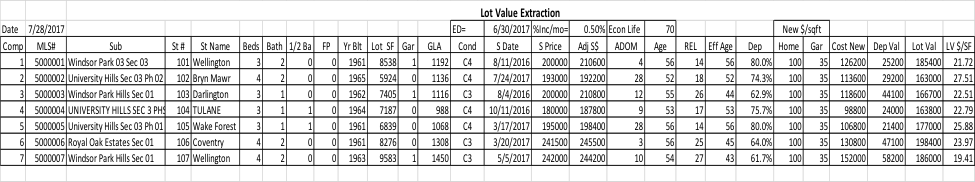Wednesday , 8 April 2020# A Spreadsheet Solution for Land Value Extraction

When the cost approach is needed, either by client requirement or applicability, it’s necessary to derive an estimate of the land value.  Of course, the best way to determine an estimate of land value is through the use of the sales comparison approach.  If there have been recent, similar lot sales in the subject’s market area, the process is relatively straightforward. It starts with defining “similar”:  size, view, location, and other amenities, such as waterfront etc.  If there are a reasonable number of comparable sales that result from a search using the appropriate criteria for similarity over a one-year period, that’s great.  If not, the time period and/or market area can be expanded as long as the sales prices are adjusted to reflect market trends and the market area expansion is into a similar neighborhood.

But, what is a “reasonable” number of comparable sales?  I normally look for at least six comparable sales to consider by analyzing lot sale price per square foot vs lot size, then use the power function to calculate the relationship between the two. The resulting formula is then used to calculate the subject’s estimated land value.  As an additional benefit, the formula can be used to calculate lot size adjustments to the comparable improved property sales included in the sales comparison grid.

Unfortunately, there are not always enough comparable sales of vacant land to permit the use of the sales comparison approach.  When this happens, a possible solution is to use the extraction method.

Using this approach, improved properties with lots comparable to the subject’s lot are identified.  In order to complete this step, the value of the improvements is calculated using the cost approach to estimate the replacement cost new.  Using effective age and remaining economic life estimates to calculate the depreciated value of the improvements, then subtracting the depreciated value of the improvements from the sales price will produce a viable land value. This is done for several properties to produce the relationship between lot value per square foot and lot size as described above for the purpose of calculating the subject’s land value and lot size adjustments used in the sales comparison grid.

There is a lot of “estimating” involved with this approach, so it works best when the value of the improvements are a low percentage of the sales price so that inaccuracies with the estimates have minimal impact on the lot value estimate.  This strategy includes selecting properties with small, older homes that have not been remodeled and with minimal site improvements.  Of course, the sales should be recent and in the subject’s neighborhood and the lots should be as similar (views, topography etc.) to the subject’s lot as possible.

The first step in the process is to search MLS using the developed criteria to produce a list of potential improved property sales that contains information such as bed and bath counts, year built, garage spaces, GLA, sales price and sale date.  Using this information, the next step is to select the “best” sales to use for the statistical analysis.  At least six should be selected. The selected sales can, then be imported into an Excel spreadsheet similar to that shown for calculating their lot value and lot value per square foot.

The MLS sheets and their descriptions and photographs should be reviewed to determine the condition of the improvements. Based upon the condition rating, age and total economic life of the improvements, the spreadsheet can be set to calculate the effective age, remaining economic life and percent depreciation.  The cost new is calculated based upon GLA, garage spaces, and unit prices. The cost new is reduced by the calculated amount of depreciation to produce the depreciated improvement value.

The improved sales prices are adjusted for date of sale, based upon the effective date of the appraisal, the estimated monthly price trend percentage for existing homes in the subjects market area, and the comparables’ dates of sale.  For each of the selected property sales, the estimated lot value (Lot Val) is calculated as the difference between the adjusted improved sales price (Adj S\$) and the depreciated value (Dep Val) of the improvements:  Lot Val = Adj S\$ – Dep ValFinally, this information is used to estimate the value of the subject lot and the adjustments to the lot sizes for the comparable properties on the sales comparison grid.  In the attached example, the Lot Value/SQFT and the Lot Acres are plotted and analyzed using regression analysis with the Power trend line. The equation is shown on the graph along with the square of the correlation coefficient. In this example, the equation is y = 7.476x-.650 where y is the lot value/sqft and x is the lot size in acres.   The Subject’s lot size is .175 acres:   y = 7.476 x .175^-.650 = \$23.215/sqft.  \$23.215/sqft x .175 acres x 43560 sqft/acre = \$176,966.In my experience, the exponent normally falls between -.4 and -.9 with a minimum R2 of about 0.3.  If there are “outliers” impacting these values, they should be removed. This process, if used sparingly, should produce reasonable coefficients and results.  In summary, if it is necessary to use the Extraction method, using the process outlined above will help to produce credible and defensible results.